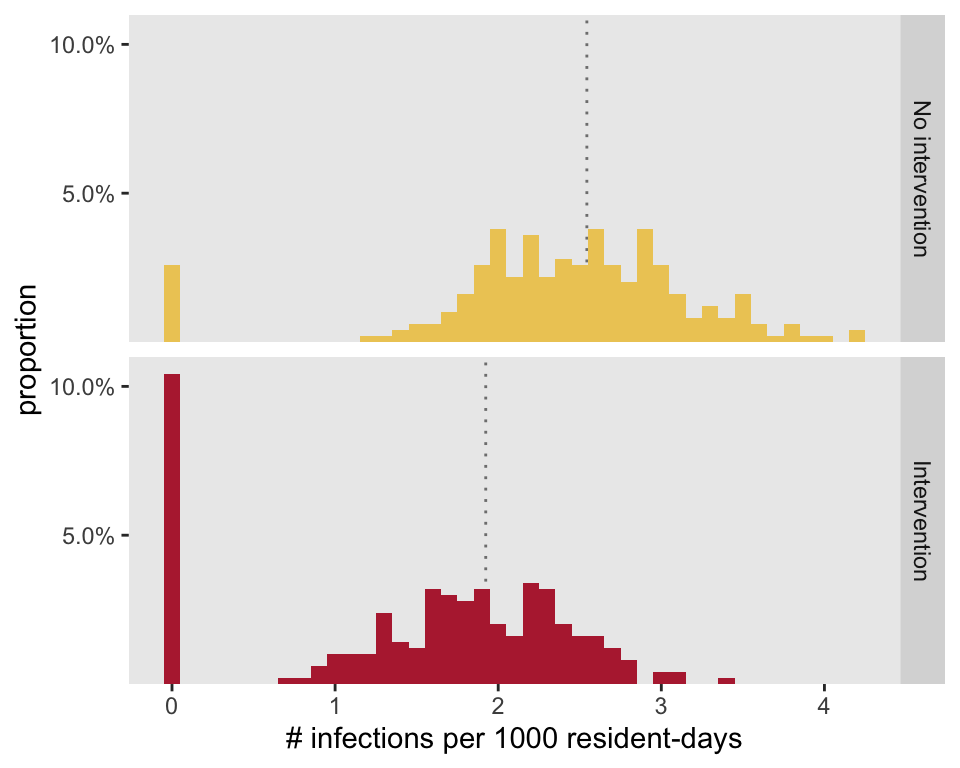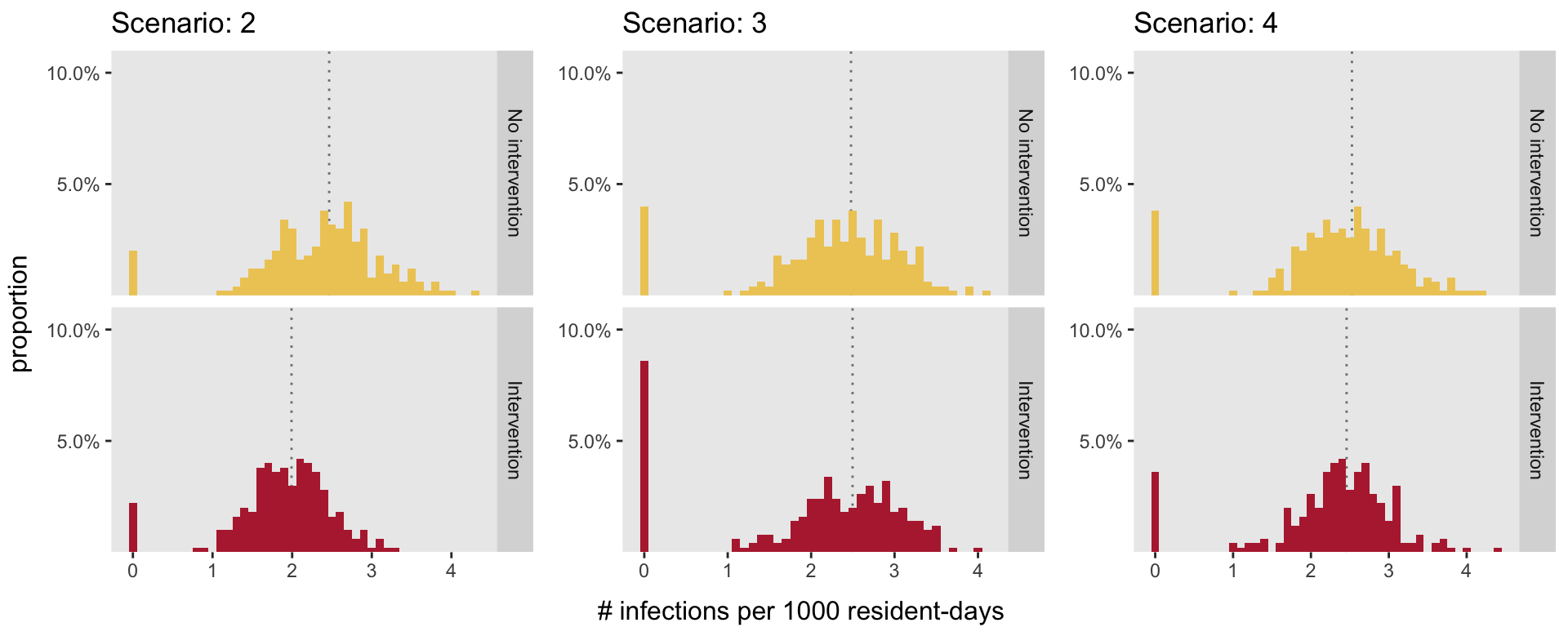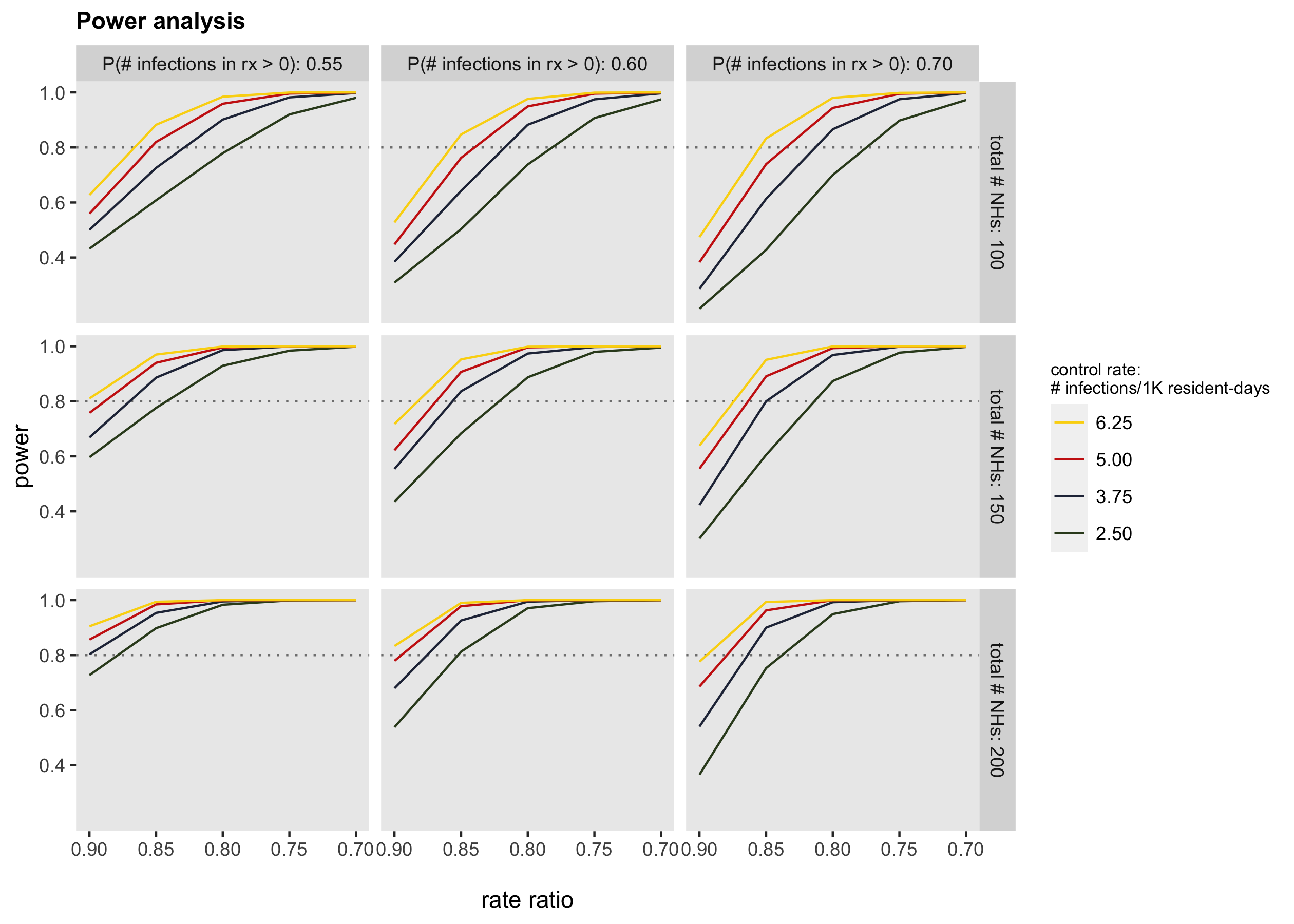# A hurdle model for COVID-19 infections in nursing homes

Late last year, I added a mixture distribution to the simstudy package, largely motivated to accommodate zero-inflated Poisson or negative binomial distributions. (I really thought I had added this two years ago - but time is moving so slowly these days.) These distributions are useful when modeling count data, but we anticipate observing more than the expected frequency of zeros that would arise from a non-inflated (i.e. “regular”) Poisson or negative binomial distribution.

There is a related, though subtly different model - the hurdle model - that I want to talk about here, because it has come up in the context of planning a new study to address COVID-19 infection rates in nursing homes using much more aggressive testing strategies.

A hurdle model is a modified count model that also assumes a relatively high frequency of zeros, but is set up as a two-stage data generation process rather than as a mixture distribution. In the first stage, the development of any (i.e. at least 1) new infections during the observation period is described by binomial probability distribution. If there is at least one infection, the process has transitioned over the “hurdle” into the second stage; here, the number of new infections is described by a non-zero count distribution, such as a truncated Poisson or truncated negative binomial distribution.

### Model specification

If $$N_i$$ is the number of new infections in nursing home $$i$$ over the observation period and we have two intervention arms $$T_i \in \{0,1\}$$, the intervention effect at each stage of the process can be modeled simply as:

$\text{logodds}\left[ P(N_i > 0) \right] = \beta_0 + \beta_1 T_i + \boldsymbol{X_i \beta_2}$

$\text{log}(N_i | N_i \ge 1) = \alpha_0 + \alpha_1 T_i + \boldsymbol{X_i \alpha_2} + \text{log}(D_i)$

The intervention effect for the binomial stage is $$\beta_1$$ (on the logodds scale) and the intervention effect for the hurdle (count) stage is $$\alpha_1$$ (on the log scale). $$\boldsymbol{X_i}$$ are any covariates that are used for stratified randomization.

$$D_i$$ is the number of resident-days observed during the follow-up period, and $$\text{log}(D_i)$$ is the “offset”; we are effectively modeling a rate of infections $$\text{log}(N_i/D_i)$$. This will take into account the fact that residents will be observed for different lengths of time - some moving into the nursing home after the study has started, and others leaving or dying before the study is complete.

### Simulating a hurdle model

Simulating data from this model is relatively straightforward, complicated only by the need to generate varying observation periods. Essentially, we must generate two outcomes - a binary outcome and a non-zero count outcome (in this case it will be from a non-zero Poisson distribution), and the observed outcome is 0 if the binary outcome is actually 0, and the value of the count outcome if the binary outcome is 1.

To get things going, here are the packages I will use. The pscl package provides a function hurdle to estimate the model parameters from our simulated data, and stargazer package outputs the model in a nice, readable format.

library(simstudy)
library(data.table)
library(ggplot2)
library(pscl)
library(stargazer)

#### Data generation

In this simulation the average observation time is 80 days (out of 90 maximum), and on average, each nursing home will have 100 residents. In the control arm, 95% of the nursing homes will have at least one infection, and 80% of the intervention arm will have at least one. The corresponding odds ratio is $$(0.80/0.20)/(.95/0.05) = 0.21$$.

The infection rate per 1000 resident-days for the control arm will be $$\sim (20/8000)*1000 = 2.5$$; for the intervention arm, the rate will be $$\sim (20/8000) *0.8 * 1000 = 2.0$$.

Here is the data definition table defHurdle created by the function defDataAdd that encodes these assumptions:

##    varname                             formula variance          dist     link
## 1:    nRes                                 100        0       poisson identity
## 2:   aDays                                  80        0       poisson identity
## 3:   nDays                     pmin(90, aDays)        0     nonrandom identity
## 4:   pDays                        nRes * nDays        0     nonrandom identity
## 5:    xBin                    0.95 - 0.15 * rx        0        binary identity
## 6:    xCnt log(20/8000)+log(0.8)*rx+log(pDays)        0 noZeroPoisson      log
## 7:       y                         xBin * xCnt        0     nonrandom identity

The data generation is only at the nursing home level. In this example, we are assuming 500 nursing homes:

set.seed(29211)
dx <- genData(500)
dx <- trtAssign(dx, grpName = "rx")

dx
##       id rx nRes aDays nDays pDays xBin xCnt  y
##   1:   1  1  113    86    86  9718    1   16 16
##   2:   2  0   89    66    66  5874    1   16 16
##   3:   3  1   83    82    82  6806    1   13 13
##   4:   4  0   91    95    90  8190    1   27 27
##   5:   5  1   97    70    70  6790    0   17  0
##  ---
## 496: 496  1  116    85    85  9860    0   17  0
## 497: 497  1   89    94    90  8010    1   14 14
## 498: 498  0  112    92    90 10080    1   20 20
## 499: 499  1   97    71    71  6887    1   21 21
## 500: 500  0   92    68    68  6256    1   13 13

#### Data visualization

A plot of the data shows the effect at each stage of the hurdle process:

dx[, rate1000 := (y/pDays)*1000]
dx[ , rx := factor(rx, labels = c("No intervention", "Intervention"))]
dm <- dx[rate1000 != 0, .(mu = mean(rate1000)), keyby = rx]

ggplot(data = dx, aes(x = rate1000)) +
geom_vline(aes(xintercept = mu), data = dm, lty = 3, color = "grey50") +
geom_histogram(binwidth = .1,
aes(y = (..count..)/sum(..count..), fill = rx)) +
facet_grid(rx ~ .) +
theme(panel.grid = element_blank(),
legend.position = "none") +
scale_y_continuous(labels = scales::percent,
name = "proportion",
expand = c(0, 0),
breaks = c(c(.05, .10)),
limits = c(0, .11)) +
scale_x_continuous(name = "# infections per 1000 resident-days") +
scale_fill_manual(values = c("#EDCB64", "#B62A3D"))#### Parameter estimation

I fit two models here. The first includes a possible intervention effect, and the second assumes no intervention effect. The purpose in fitting the second model is to provide a basis of comparison.

hfit1 <- hurdle(y ~ rx | rx, offset = log(pDays), data = dx)
hfit1.0 <- hurdle(y ~ 1 | 1, offset = log(pDays), data = dx)

The hurdle model returns two sets of estimates. The first component of the model shown here is binomial model. The estimated intervention effect (odds ratio) is $$exp(-1.570) = 0.21$$, as expected. Note that the log-likelihood reported here is for the composite hurdle model (both stages).

stargazer(hfit1, hfit1.0, type = "text", zero.component = TRUE,
notes =" ", notes.append = FALSE, notes.label="",
dep.var.labels.include = FALSE, dep.var.caption = "",
omit.stat = "n", object.names = TRUE, model.numbers = FALSE)
##
## ====================================
##                  hfit1     hfit1.0
## ------------------------------------
## rxIntervention -1.570***
##                 (0.325)
##
## Constant        2.900***   1.900***
##                 (0.285)    (0.133)
##
## ------------------------------------
## Log Likelihood -1,424.000 -1,511.000
## ====================================
## 

The second component is the count model. The estimated intervention effect is $$exp(-0.279) = 0.76$$, which is close to the true value of $$0.80$$. (The reported log-likelihoods are the same as in the binomial model.)

stargazer(hfit1, hfit1.0, type = "text", zero.component = FALSE,
notes =" ", notes.append = FALSE, notes.label="",
dep.var.labels.include = FALSE, dep.var.caption = "",
omit.stat = "n", object.names = TRUE, model.numbers = FALSE)
##
## ====================================
##                  hfit1     hfit1.0
## ------------------------------------
## rxIntervention -0.279***
##                 (0.023)
##
## Constant       -5.980***  -6.090***
##                 (0.014)    (0.011)
##
## ------------------------------------
## Log Likelihood -1,424.000 -1,511.000
## ====================================
## 

In this particular case, the intervention alters both the binomial probability and the county distribution, but that will not necessarily always be the case. A log-likelihood ratio test (LRT) is a global test that compares the model that explicitly excludes an intervention effect (hfit1.0) with the model that includes an intervention effect. If the likelihoods under each are close enough, then the model that excludes the intervention effect is considered sufficient, and there is no reason to conclude that the intervention is effective. We can use the p-value based on the LRT as a measure of whether or not the intervention is generally effective, either because it changes the binomial probability, the count distribution, or both.

In this case, the p-value is quite low:

lrt1 <- -2*(logLik(hfit1.0) - logLik(hfit1))
1 - pchisq(lrt1, 2)
## 'log Lik.' 0 (df=2)

### Alternative scenarios

Here are three additional scenarios that provide examples of ways the intervention can affect the outcome. In Scenario 2, the intervention no longer has an effect on the probability of having at least one infection, but still has an effect on the count. In Scenario 3, the intervention only effects the probability of having at least one infection, and not the count distribution. And in Scenario 4, the intervention has no effect at all at either stage.

defHurdle.V2 <- updateDef(defHurdle, "xBin", "0.95")
defHurdle.V3 <- updateDef(defHurdle, "xCnt", "log(20/8000) + log(pDays)")
defHurdle.V4 <- updateDef(defHurdle.V3, "xBin", "0.95")

The plots bear out the underlying parameters. We can see the probability of a zero is the same across treatment arms in Scenario 2, just as the distributions of the count variable in Scenario 3 appear equivalent. In Scenario 4, it is hard to distinguish between the two distributions across interventions.Here are the model fits - the results are consistent with the plots:

##
## ================================================================================
##                  hfit2     hfit2.0     hfit3     hfit3.0     hfit4     hfit4.0
## --------------------------------------------------------------------------------
## rxIntervention   -0.099              -0.871***               0.058
##                 (0.446)               (0.287)               (0.342)
##
## Constant        3.180***   3.130***   2.440***   1.940***   2.500***   2.530***
##                 (0.323)    (0.223)    (0.233)    (0.135)    (0.239)    (0.171)
##
## --------------------------------------------------------------------------------
## Log Likelihood -1,443.000 -1,489.000 -1,458.000 -1,463.000 -1,463.000 -1,464.000
## ================================================================================
## 
##
## ================================================================================
##                  hfit2     hfit2.0     hfit3     hfit3.0     hfit4     hfit4.0
## --------------------------------------------------------------------------------
## rxIntervention -0.210***               0.010                 -0.029
##                 (0.022)               (0.022)               (0.021)
##
## Constant       -6.010***  -6.110***  -6.000***  -6.000***  -5.980***  -6.000***
##                 (0.015)    (0.011)    (0.015)    (0.011)    (0.015)    (0.010)
##
## --------------------------------------------------------------------------------
## Log Likelihood -1,443.000 -1,489.000 -1,458.000 -1,463.000 -1,463.000 -1,464.000
## ================================================================================
## 

And finally, the p-values from the LRTs of the models under each of the three scenarios are consistent with the underlying data generating processes. It is only in the last scenario where there is no reason to believe that the intervention has some sort of effect.

round(c(lrt2 = 1 - pchisq(lrt2, 2),
lrt3=1 - pchisq(lrt3, 2),
lrt4=1 - pchisq(lrt4, 2)), 4)
##   lrt2   lrt3   lrt4
## 0.0000 0.0067 0.3839

This work was supported in part by the National Institute on Aging (NIA) of the National Institutes of Health under Award Number U54AG063546, which funds the NIA IMbedded Pragmatic Alzheimer’s Disease and AD-Related Dementias Clinical Trials Collaboratory (NIA IMPACT Collaboratory). The author, a member of the Design and Statistics Core, was the sole writer of this blog post and has no conflicts. The content is solely the responsibility of the author and does not necessarily represent the official views of the National Institutes of Health.

If you’ve visited my blog before, you might have picked up on the fact that I like to use simulation to estimate sample size or power when planning a randomized trial. This allows me to be sure everyone understands the assumptions.

To estimate power, I generate multiple data sets under a specific set of assumptions and estimate intervention effects for each data set. The power of the study under this set of assumptions is the proportion of times we would conclude that the intervention is effective. In the context of a hurdle model, I use the p-value from the LRT as the arbiter of effectiveness; the proportion of p-values less than 0.05 is the power.

gData <- function(n, def) {

dx <- genData(n)
dx <- trtAssign(dx, grpName = "rx")

dx[]

}

estModel <- function(dx) {

hfit <- hurdle(y ~ rx | rx, offset = log(pDays), data = dx, )
hfit0 <- hurdle(y ~ 1 | 1, offset = log(pDays), data = dx)
lrt <- -2*(logLik(hfit0) - logLik(hfit))

data.table(p.zero = coef(summary(hfit))$zero["rx", "Pr(>|z|)"], p.count = coef(summary(hfit))$count["rx", "Pr(>|z|)"],
X2 = 1 - pchisq(lrt, 2))

}

iter <- function(n, defHurdle, i) {

dx <- gData(n, def)
hfit <- estModel(dx)
return(data.table(i = i, hfit))

}

diter <- rbindlist(lapply(1:1000, function(i) iter(50, defHurdle, i)))

Here are the results from the individual replications Scenario 1 effect assumptions and 50 nursing homes:

diter
##          i p.zero  p.count       X2
##    1:    1 0.9975 4.06e-04 0.000437
##    2:    2 0.0449 1.05e-03 0.000216
##    3:    3 0.0713 5.92e-03 0.002246
##    4:    4 0.0449 5.85e-04 0.000128
##    5:    5 0.1891 3.20e-02 0.034025
##   ---
##  996:  996 0.3198 7.04e-03 0.014600
##  997:  997 0.1891 1.13e-02 0.013579
##  998:  998 0.3198 8.16e-04 0.001973
##  999:  999 1.0000 4.45e-06 0.000023
## 1000: 1000 0.5590 2.34e-03 0.007866

And here is the estimate of power - in this case there is about 90% power that we will conclude that there is an effect of some type given the assumptions under Scenario 1:

diter[, mean(X2 <= 0.05)]
##  0.898

In conclusion, here is a power plot for a range of effect size assumptions, sample size assumptions, and control arm assumptions. In all of these cases, I assumed that the binomial probability under the control condition would be 70%, (If anyone wants to see the code for generating all of this data and the plot, I can post on github. However, it is really just an extension of what is shown here.)